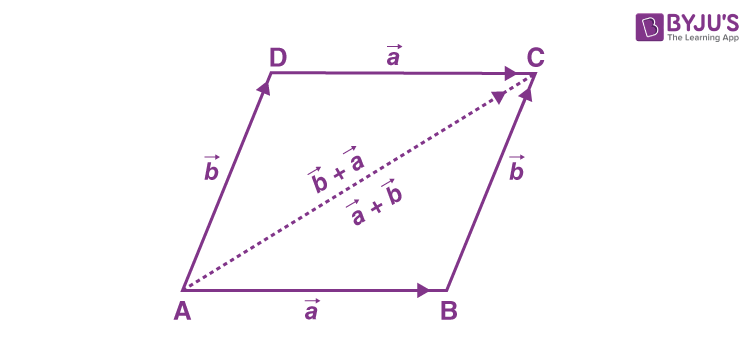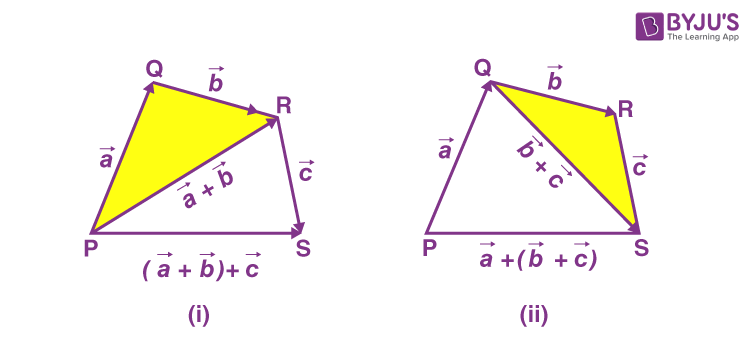A vector is a quantity that has both direction and magnitude. A vector is a directed line segment which is denoted by $$\overline{AB}$$ or $$\vec{a}$$. The arrows represent the direction of the vector. The point A where the $$\vec{a}$$ starts is called the initial point and the point B where the $$\vec{a}$$ ends is called the terminal point. The distance between the initial and terminal point of the vector AB is called the length or the magnitude of a vector and it is represented by “a” or $$|\vec{a}|$$ or $$|\overline{AB}|$$. Also, the length or the magnitude of a vector cannot be negative. In this article, we are going to learn the two important properties of vector addition in detail.

## Two Properties of Vector Addition

We know that the vector addition is the sum of two or more vectors. Two important laws associated with vector addition are triangle law and parallelogram law. Similarly, the properties associated with vector addition are:

• Commutative Property
• Associative Property

Now, let us discuss the two properties of vector addition in detail.

### Commutative Property of Vector Addition

The commutative property of vector addition states that “For any two vectors, $$\vec{a}$$ and $$\vec{b}$$, $$\vec{a}$$ + $$\vec{b}$$ = $$\vec{b}$$+ $$\vec{a}$$ ”.

Proof:

Consider a parallelogram ABCD as shown in the figure.Let $$|\overline{AB}|$$ = $$\vec{a}$$ and $$|\overline{BC}|$$ = $$\vec{b}$$.

Now, by using the triangle law of vector addition from the triangle ABC, we can write:

$$\overline{AC}=\vec{a}+\vec{b}$$

Since, the opposite sides of a parallelogram are parallel and equal, we have $$|\overline{AD}|$$ = $$|\overline{BC}|$$ = $$\vec{b}$$.

Now, again use the triangle law from the triangle ADC, we get

$$|\overline{AC}|$$ = $$|\overline{AD}|$$ + $$|\overline{DC}|$$=$$\vec{b}$$+$$\vec{a}$$.

Therefore, $$\vec{a}+\vec{b}$$ = $$\vec{b}+\vec{a}$$.

Hence, the commutative property of vector addition is proved.

### Associative Property of Vector Addition

The associative property of vector addition states that “for any three vectors, $$\vec{a}$$, $$\vec{b}$$ and $$\vec{c}$$, $$(\vec{a}+\vec{b})+\vec{c}= \vec{a}+(\vec{b}+\vec{c})$$”.

Proof:

Assume the vectors $$\vec{a}$$, $$\vec{b}$$ and $$\vec{c}$$ are represented by $$\overline{PQ}$$, $$\overline{QR}$$, and $$\overline{RS}$$ as shown in the figure.Thus,

$$\vec{a}+\vec{b} = \overline{PQ}+\overline{QR} = \overline{PR}$$

Similarly,

$$\vec{b}+\vec{c} = \overline{QR}+\overline{RS} = \overline{QS}$$

Hence,

$$(\vec{a}+\vec{b})+\vec{c} = \overline{PR}+\overline{RS} = \overline{PS}$$, and

$$\vec{a}+(\vec{b}+\vec{c}) = \overline{PQ}+\overline{QS} = \overline{PS}$$

(i.e) $$(\vec{a}+\vec{b})+\vec{c}= \vec{a}+(\vec{b}+\vec{c})$$

Therefore, the associative property of vector addition is proved.

Stay tuned with BYJU’S – The Learning App and download the app to learn all Maths-related concepts by exploring more videos.